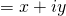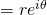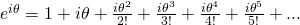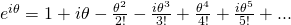# Deriving the polar form for complex numberI always stress to students the importance of the basics of complex numbers, that isThe three forms of the complex numbers. I have shown how to derive from the cartesian () to trigonometric form () form. But I seldom show students how to derive the polar form due to the rigor. So here we go. Lets first identify a few formulas from MF15.Let us consider the polar form then.Resolving theRearranging themNotice if we factoriseoutThen with the formula we started with,Voila! We are done and we have successfully derive our trigonometric form.

Not readable? Change text.